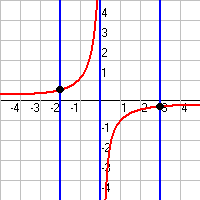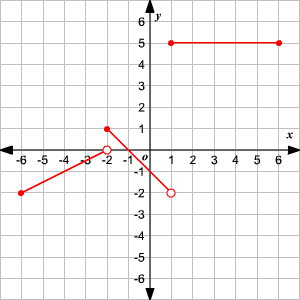# The Vertical Line Test

You can check whether a graph represents a function by using the vertical line test.

The test states that a graph represents a function if and only if all vertical lines intersect the graph at most once.

Example :

 This graph does not represent a function: for example, the vertical line $x=1$ intersects it in three places. This graph does represent a function, since any vertical line intersects the graph exactly once, except $x=0$ , which doesn't intersect it at all.Consider the graph shown.You can see that no vertical lines pass through two points on the graph.

Note that $\left(-2,0\right)$ and $\left(1,-2\right)$ are open points and are not on the graph. So, the vertical lines $x=-2$ and $x=1$ pass through only one point on each of the line segments.

Therefore, the graph shown is of a function.

The step function can be defined as:

$\begin{array}{ccc}\begin{array}{l}y=\frac{1}{2}x+1\\ y=-x-1\\ y=5\end{array}& \begin{array}{l}\\ \\ \end{array}& \begin{array}{l}-6\le x<-2\\ -2\le x<1\\ \text{\hspace{0.17em}}\text{\hspace{0.17em}}\text{\hspace{0.17em}}1\le x\le 6\end{array}\end{array}$

The domain of the function is $-6\le x\le 6$ and the range is $-2\le y\le 5$ .